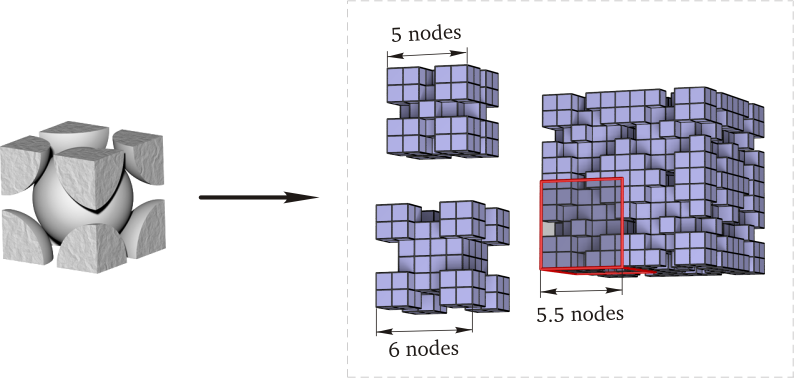Siarhei Khirevich's site

# MATLAB code for discretization of regular sphere packings

Here you can find the matlab scripts used for discretization of regular sphere packings (of BCC, FCC, and SC types) in the work I co-authored. Here "discretization" means mapping of a geometry with analytical description onto a uniform cubic lattice:The purpose of scripts is to reduce a scatter of output transport coefficients, drag force or permeability. The main routine is pack_discretization.m with documentation located in its comments. This routine calls binary2vector.m for initialization of sphere coordinates and calc_rad_scal_factor.m calculating radius for a given porosity value.

An optional routine isBoxBad.m helps to check the ratio between the edge E of a lattice and the number of unit cells U to be replicated along each Cartesian direction — both are input parameters for pack_discretization.m. If isBoxBad.m returns one for a given pair (E, U), other value of E should be taken, otherwise results of a flow simulation for the given lattice edge E can be obtained using smaller number of unit cells.